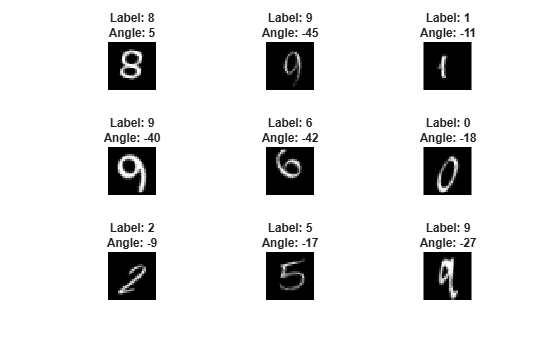## Make Predictions Using `dlnetwork` Object

This example shows how to make predictions using a `dlnetwork` object by splitting data into mini-batches.

For large data sets, or when predicting on hardware with limited memory, make predictions by splitting the data into mini-batches. When making predictions with `SeriesNetwork` or `DAGNetwork` objects, the `predict` function automatically splits the input data into mini-batches. For `dlnetwork` objects, you must split the data into mini-batches manually.

### Load `dlnetwork` Object

Load a trained `dlnetwork` object and the corresponding classes.

```s = load("digitsCustom.mat"); dlnet = s.dlnet; classes = s.classes;```

Load the digits data for prediction.

```digitDatasetPath = fullfile(matlabroot,'toolbox','nnet','nndemos', ... 'nndatasets','DigitDataset'); imds = imageDatastore(digitDatasetPath, ... 'IncludeSubfolders',true);```

### Make Predictions

Loop over the mini-batches of the test data and make predictions using a custom prediction loop. To read a mini-batch of data from the datastore, set the `ReadSize` property to the mini-batch size.

For each mini-batch:

• Convert the data to `dlarray` objects with underlying type single and specify the dimension labels `'SSCB'` (spatial, spatial, channel, batch).

• For GPU prediction, convert to `gpuArray` objects.

• Make predictions using the `predict` function.

• Determine the class labels by finding the maximum scores.

Specify the prediction options. Specify a mini-batch size of 128 and make predictions on a GPU if one is available. Using a GPU requires Parallel Computing Toolbox™ and a CUDA® enabled NVIDIA® GPU with compute capability 3.0 or higher.

```miniBatchSize = 128; executionEnvironment = "auto";```

Set the read size property of the image datastore to the mini-batch size.

`imds.ReadSize = miniBatchSize;`

Make predictions by looping over the mini-batches of data.

```numObservations = numel(imds.Files); YPred = strings(1,numObservations); i = 1; % Loop over mini-batches. while hasdata(imds) % Read mini-batch of data. data = read(imds); X = cat(4,data{:}); % Normalize the images. X = single(X)/255; % Convert mini-batch of data to dlarray. dlX = dlarray(X,'SSCB'); % If training on a GPU, then convert data to gpuArray. if (executionEnvironment == "auto" && canUseGPU) || executionEnvironment == "gpu" dlX = gpuArray(dlX); end % Make predictions using the predict function. dlYPred = predict(dlnet,dlX); % Determine corresponding classes. [~,idxTop] = max(extractdata(dlYPred),[],1); idxMiniBatch = i:min((i+miniBatchSize-1),numObservations); YPred(idxMiniBatch) = classes(idxTop); i = i + miniBatchSize; end```

Visualize some of the predictions.

```idx = randperm(numObservations,9); figure for i = 1:9 subplot(3,3,i) I = imread(imds.Files{idx(i)}); label = YPred(idx(i)); imshow(I) title("Label: " + label) end```